Disclosure: This post may contain affiliate links, meaning when you click the links and make a purchase, we receive a commission.

# For Next Loop in VBA Excel (How to Step and Exit Loop)

In this article, I shall discuss at first how to use For Next Loop in Excel VBA. Then I will elaborate on the discussion on how to exit a For Loop when some condition is there and then you will learn how to step up or step down For Next Loop VBA Excel.

So, let’s start…

Generally, people don’t like to do repetitive works. Right? If you’re a really creative person, doing the same tasks, again and again, is really tough for you. In every programming language, there are Loops to do some kind of repetitive works. Excel VBA is no exception.

Today, we are going to discuss one of the Excel Loops, For Next Excel VBA Loop.

## How to use For Next Loop VBA Excel

Say, you want to find the summation of square numbers from 1 to 10.

I mean, we want this: Total = 12+ 22 + 32 + 42 + … …. … + 102

The following code will do this thing for you.

We use a For-Next loop to execute one or more statements for more than one time. The following is an example of a For-Next loop:

 Source code```Sub SumOfSquaredNumber()
Total = 0
For Num = 1 To 10
Total = Total + (Num ^ 2)
Next Num
MsgBox Total
End Sub```

The following figure shows the SumOfSquaredNumber macro code and resultant pop-up dialog box showing the sum of 1 to 10 squared numbers.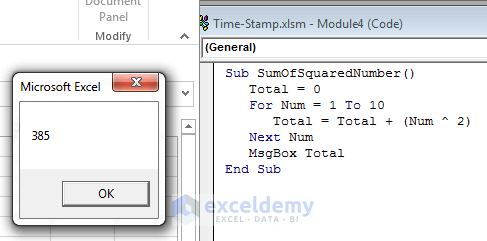The Dialog box is showing the sum of 1 to 10 squared numbers.

This macro code has one statement between the For statement and the Next statement. The statement is: Total = Total + (Num ^ 2). This single statement is executed ten times.

### How does this code work?

We have discussed every line of the above macro to make you understand how this code works. This idea is very important. You will use this For-Next loop many times in your code.

1. Sub SumOfSquaredNumber(): This statement starts the macro subprocedure.
2. Total = 0: Total is a variable. We assign value 0 in this variable with this statement.
3. For Num = 1 To 10: Num is also a variable and it is assigned now value 1. Excel checks Num’s assigned value (now, 1) with Num’s limiting value (here, 10). If Num’s assigned value is less than or equal to Num’s limiting value, then the statement between For and Next will execute.
4. Total = Total + (Num ^ 2): Num variable’s value (right now 1) will be squared here at first. Excel then sums the square value of Num (1) and the value of Total (0). The result of the sum will be assigned to Total again. Finally Total variable now holds: 1 (0+1=1).
5. Next Num: This statement increases the value of Num variable by 1. So Num’s value is now 1+1=2. Excel now checks again Num’s assigned value (2) with Num’s limiting value (10). As assigned value is less than limiting value, statement Total = Total + (Num ^ 2) executes again.
6. Total = Total + (Num ^ 2): This statement now executes again. Num variable’s value (right now 2) will be squared here at first. Excel then sums the square value of Num (4) and the value of Total (1). The result of the sum will be assigned to Total again. Finally Total variable now holds 5 (4+1=5).
7. Next Num: This statement increases the value of Num variable by 1. So Num’s value is now 2+1=3. Excel now checks again Num’s assigned value (3) with Num’s limiting value (10). As assigned value is less than limiting value, statement Total = Total + (Num ^ 2) executes again.
8. Total = Total + (Num ^ 2): This statement now executes again. Num variable’s value (right now 3) will be squared here at first. Excel then sums the square value of Num (9) and the value of Total (5). The result of the sum will be assigned to Total again. Finally Total variable now holds 14 (9+5=14).
9. In this way, statement Total = Total + (Num ^ 2) will be executed until Num’s value is equal to 10.
10. MsgBox Total: This statement shows a pop-up dialog box with the value of Total (385).

End Sub: This statement ends the macro subprocedure.

## Life Without For-Next Loop in Excel VBA

Let’s see an example.

I want to give the cells A1: A20, a light blue fill using VBA code first with a manual repetitive approach.

So, go to Developer>Controls>Insert and under the ActiveX Controls section, choose Command Button and create a command button.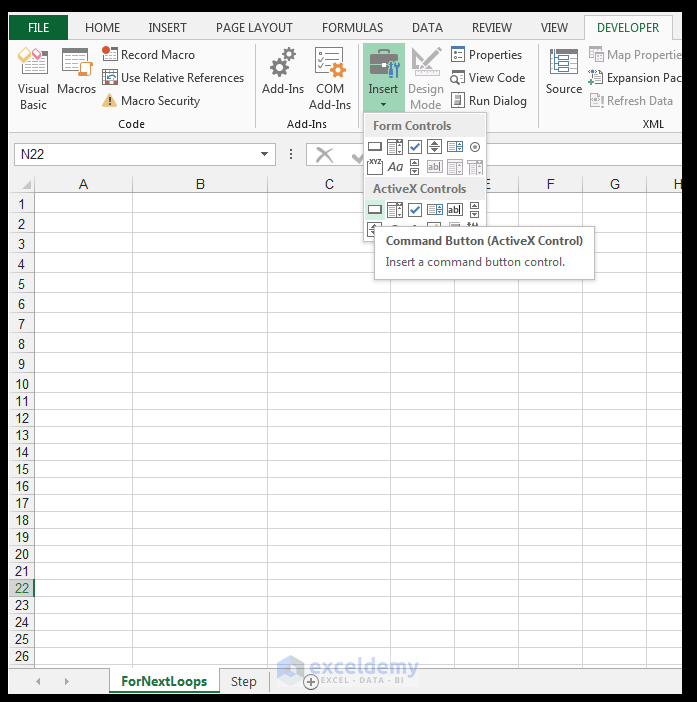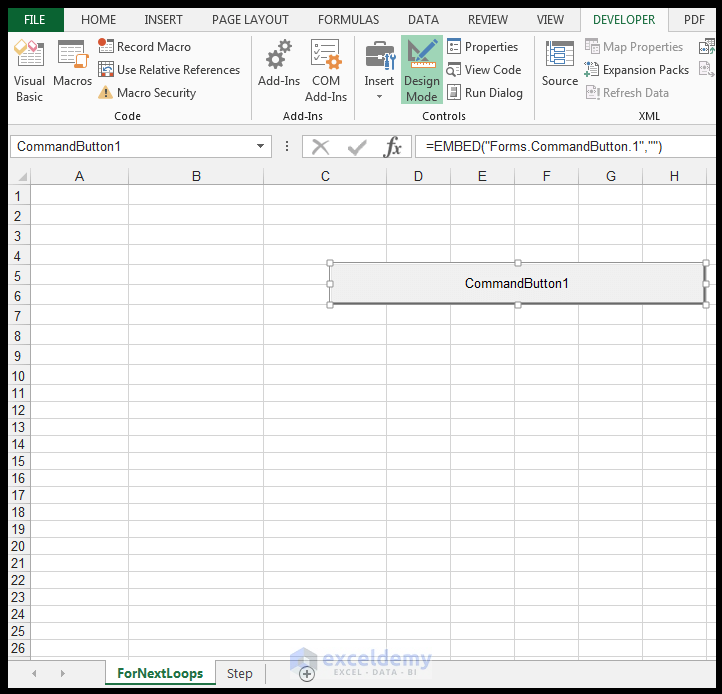Using the Properties Window, name the Command button, cmdwithoutLoop, and the caption to Fill without using a loop.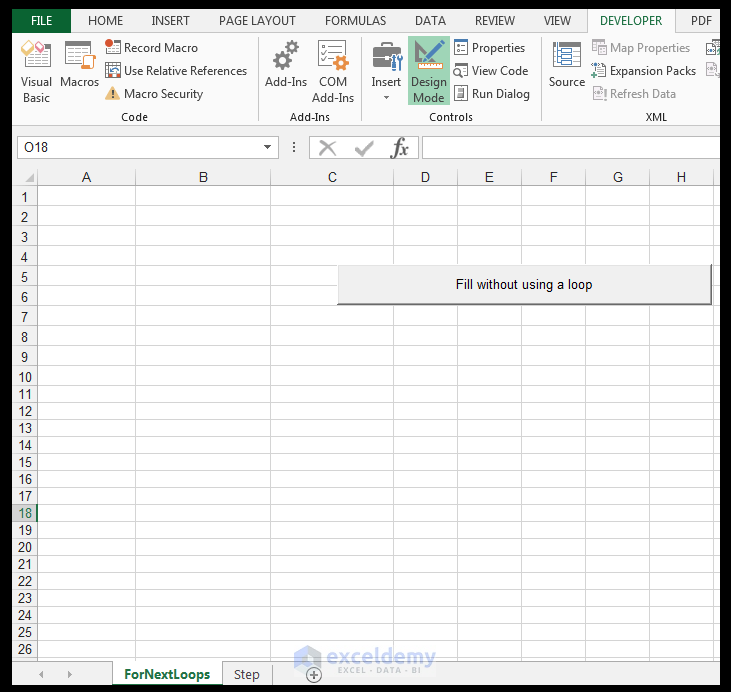Right-click the button and select View Code.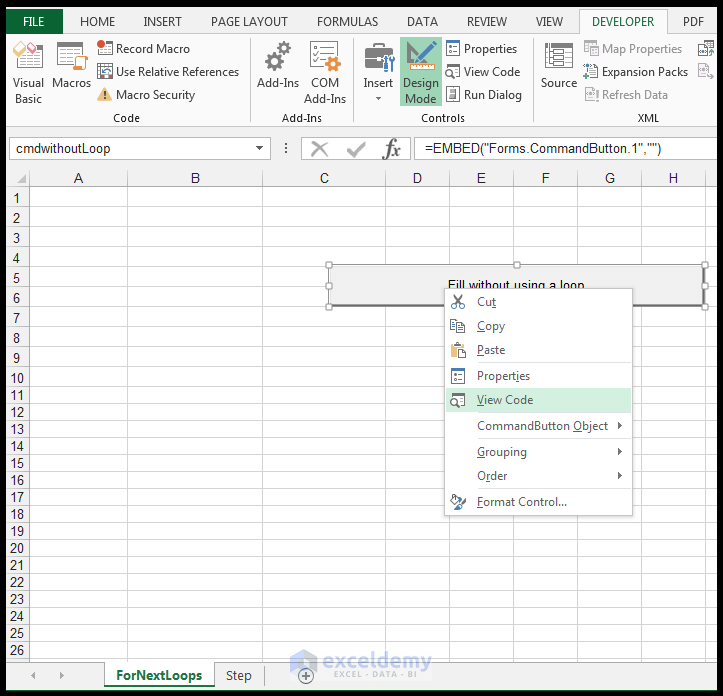Then you will see Excel VBA Editor and Insert the following code for the button click event, in order to fill the cells in range A1: A20 with a light blue fill, using a repetitive coding block.

 Source code```Private Sub cmdwithoutLoop_Click()
Cells(1, "A").Interior.Color = RGB(156, 207, 212)
Cells(2, "A").Interior.Color = RGB(156, 207, 212)
Cells(3, "A").Interior.Color = RGB(156, 207, 212)
Cells(4, "A").Interior.Color = RGB(156, 207, 212)
Cells(5, "A").Interior.Color = RGB(156, 207, 212)
Cells(6, "A").Interior.Color = RGB(156, 207, 212)
Cells(7, "A").Interior.Color = RGB(156, 207, 212)
Cells(8, "A").Interior.Color = RGB(156, 207, 212)
Cells(9, "A").Interior.Color = RGB(156, 207, 212)
Cells(10, "A").Interior.Color = RGB(156, 207, 212)
Cells(11, "A").Interior.Color = RGB(156, 207, 212)
Cells(12, "A").Interior.Color = RGB(156, 207, 212)
Cells(13, "A").Interior.Color = RGB(156, 207, 212)
Cells(14, "A").Interior.Color = RGB(156, 207, 212)
Cells(15, "A").Interior.Color = RGB(156, 207, 212)
Cells(16, "A").Interior.Color = RGB(156, 207, 212)
Cells(17, "A").Interior.Color = RGB(156, 207, 212)
Cells(18, "A").Interior.Color = RGB(156, 207, 212)
Cells(19, "A").Interior.Color = RGB(156, 207, 212)
Cells(20, "A").Interior.Color = RGB(156, 207, 212)
End Sub```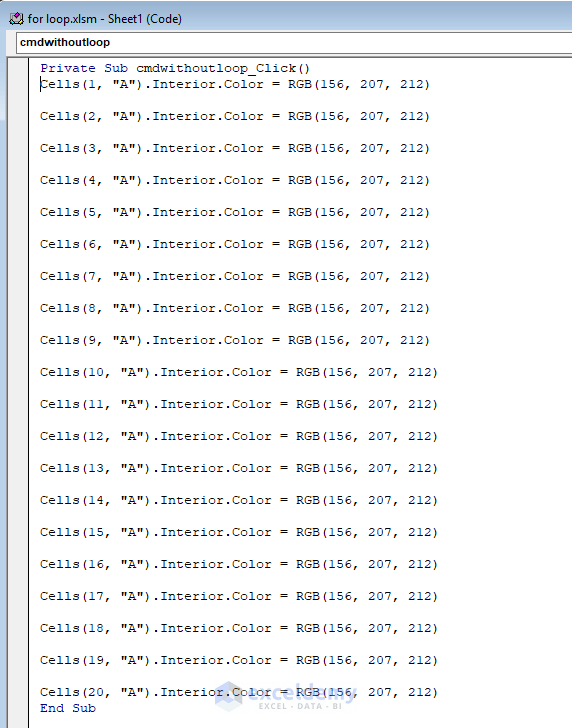Return to the worksheet and make sure Design Mode is not activated, click on the button and the cells specified in the code will now be filled with a light blue color as shown below.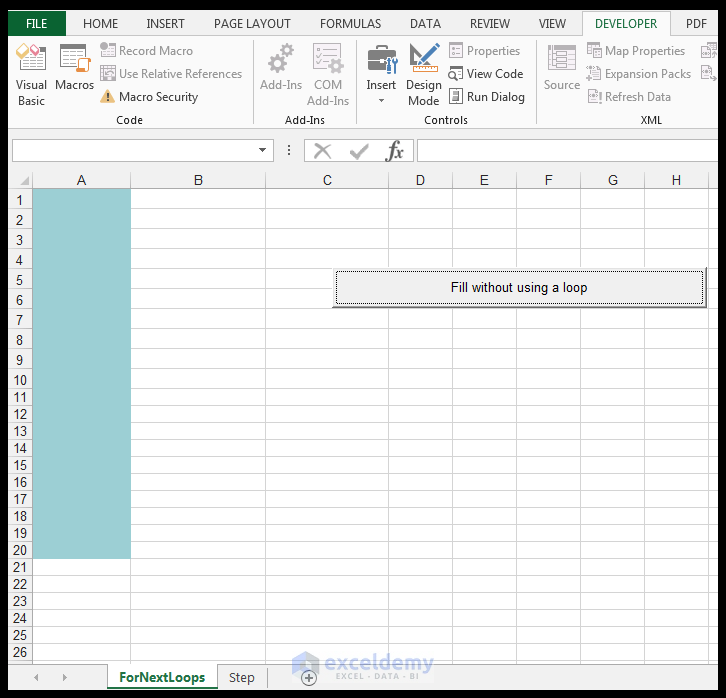## Filling the Cells with Color with For Next Loop

The For…Next Loop is very simple to use and understand.

So, let’s see how to write above code using For – Next Loop.

First, add another command button following the above way with the caption “Fill using a For Next loop”.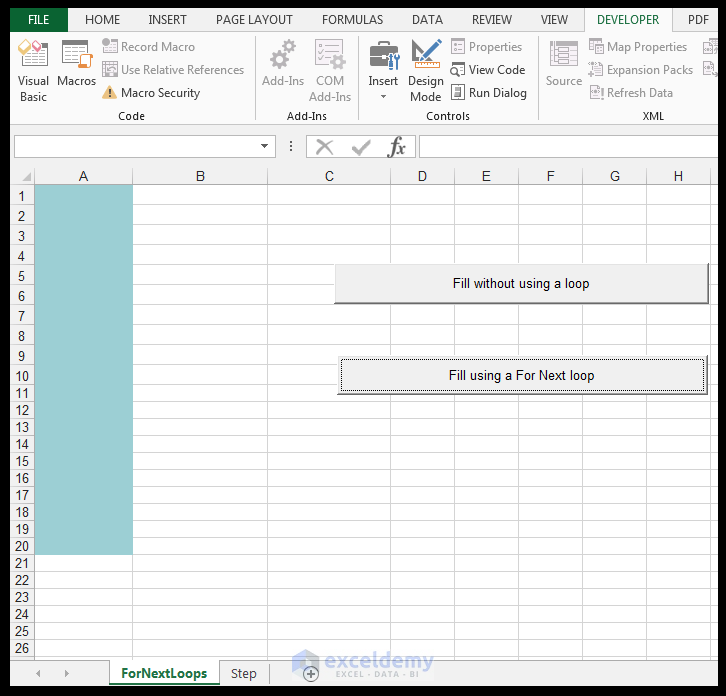Then open Excel VBA Editor and paste the following code for the button click event in order to fill the cells in the range A1: A20 with a light blue fill.

 Source code```Private Sub cmdusingLoop_Click()
Dim varcounter As Integer
For varcounter = 1 To 20
Cells(varcounter, "A").Interior.Color = RGB(156, 207, 212)
Next varcounter
End Sub```

Return back to the worksheet and make sure Design Mode is not activated click on the second button.

The cells A1: A20 are now filled with the light blue fill.

Now using For…Next Loop we have done the work very shortly and easily.

## Excel VBA For Next Loop Stepping

The Step keyword is an optional keyword that can be used in conjunction with the For…Next Loop Structure. By default without it, the For…Next Loop size is 1. When the Step value is specified, the counting variable increments by the specific step size set. The Step value can be either a positive or a negative integer. By specifying the Step value, one directly increments the counter as the loop proceeds. This concept is best demonstrated with an example.

### Using a Positive Step Value

We now would like to fill every second cell in the range A1: A20, starting on cell A1, and then moving to A3 and so on,  with a light green fill, using the For…Next Loop structure with the Step keyword, and a positive step value. In this case, our step value would be positive 2, since we are incrementing using a value of 2.

1) On the worksheet called Step, go to Developer>Controls>Insert and under the ActiveX Controls section, choose Command Button and draw a button on the worksheet.

2) Using the Properties Window, name the Command button, cmdusingloopwithStep and the caption to Fill using a For Next loop With a Positive Step Value.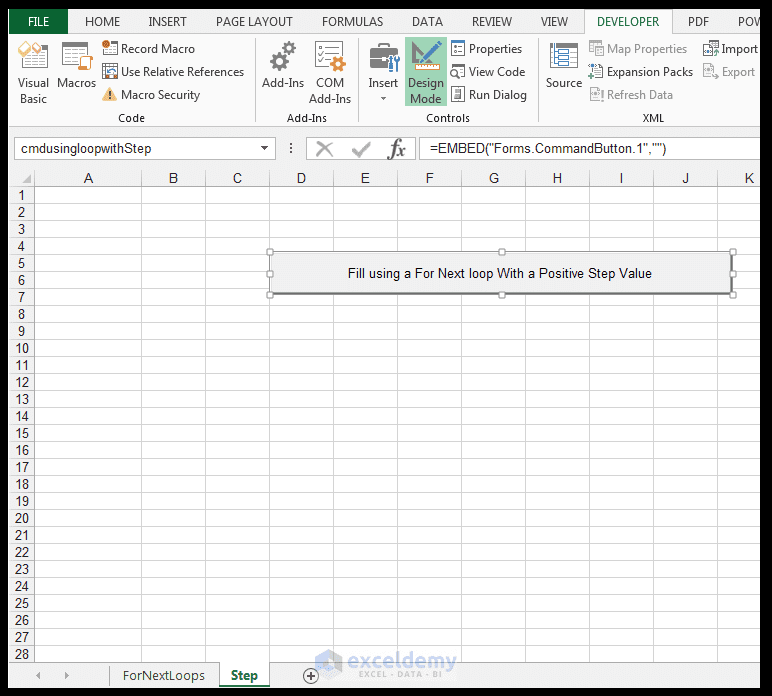3) Right-click the button and select View Code.

4) Enter the following code for the button click event in order to fill every second cell in range A1:A20 with a light green fill (we will start on cell A1 and fill this cell with the light green fill and then every second cell from there onwards will also receive the light green fill), using a For…Next Loop structure with the Step keyword.

 Source code```Private Sub cmdusingloopwithStep_Click()

Dim icounter As Integer

For icounter = 1 To 20 Step 2

Cells(icounter, "A").Interior.Color = RGB(171, 248, 128)

Next icounter

End Sub```

5) Return back to the worksheet and make sure Design Mode is not activated click on the button.

6) Every second cell in the range is now filled with the light green fill, starting with cell A1 and then moving by an increment of 2, due to us specifying using the Step keyword.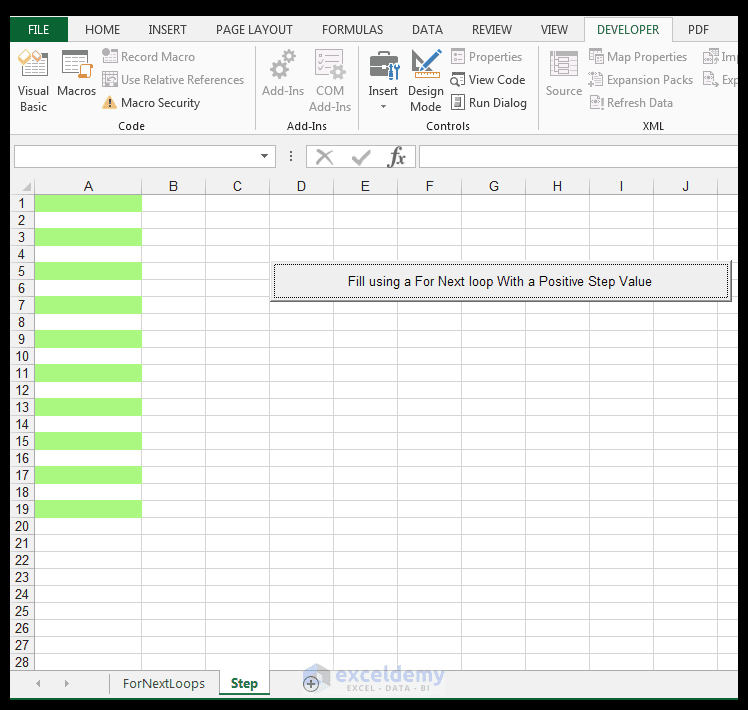### Using a Negative Step Value

We now would like to fill cells A2, A4, A6, A8, A10, A12, A14, A16, A18 and A20 with a grey fill, using the For…Next Loop structure with the Step keyword, using a negative step value. In this case, our step value is negative 2 since we are starting at cell A20 and then counting down technically due to the negative 2 value.

1) On the worksheet called Step, go to Developer>Controls>Insert and under the ActiveX Controls section, choose Command Button and draw the second button on the worksheet.

2) Using the Properties Window, name the Command button, cmd using loop with negative Step and the caption to Fill using a For Next loop With a Negative Step Value.

3) Right-click the button and select View Code.

4) Enter the following code for the button click event in order to fill the cells A2, A4, A6, A8, A10, A12, A14, A16, A18 and A20 with a grey fill, using a For…Next Loop structure with the Step keyword and a negative value.

 Source code```Private Sub cmdusingloopwithnegativeStep_Click()

Dim icountertwo As Integer

For icountertwo = 20 To 1 Step -2

Cells(icountertwo, "A").Interior.Color = RGB(173, 173, 173)

Next icountertwo

End Sub```

5) Return back to the worksheet and make sure Design Mode is not activated click on the button.

6) Every second cell in the range is now filled, starting with cell A20 and then moving 2 up, with the grey fill, due to us specifying using the Step keyword.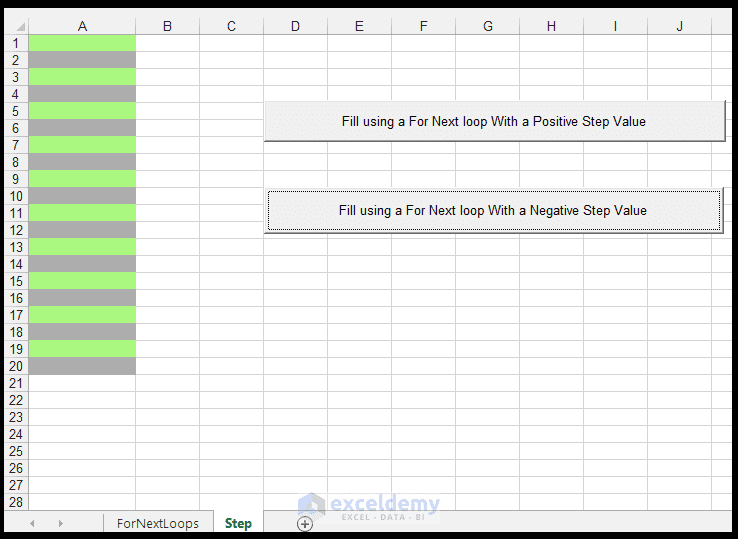7) Remember to save your workbook as a macro-enabled workbook if you haven’t done so already.

We can see when using the For…Next Loop structure with a negative Step value, the sequence is slightly different and we start counting from the highest value downwards.

## How to Exit For Next Loop

Now I want to show you, how to exit from a For-Next Loop with an example.

 Source code```Sub ExitForNext()
Dim i As Integer
For i = 1 To 10
If i = 5 Then Exit For
Next i
MsgBox i
End Sub```

When i=5, the loop will be stopped and then the value of i will show. MsgBox will create a dialog box and will show the value of variable i (shown in the following image).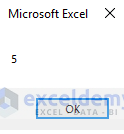LoopsForNext

## Conclusion

Looping allows one to handle repetitive actions, in an efficient manner. VBA provides different types of Loops and the simplest one is the For…Next Loop and this is used when one has a finite number of actions/iterations needing to be completed. This loop structure can also be used in conjunction with a step value which can be either positive or negative, which allows slightly more flexibility and functionality with respect to incrementing.

Please feel free to tell us if you use the For…Next Loop structure in your VBA code.

## Useful VBA related Links from ExcelDemy

How to Use the For Each Next Loop in Excel VBA

How to Use the Do While Loop in Excel VBA

## Review Section: Test your Understanding

1) What is a loop?

2) When does one use a looping structure and what are the key advantages of using loops in one’s VBA code?

3) What is the general syntax of the For…Next Loop?

4) What is the difference between using a positive Step Value versus a negative Step Value in a For…Next Loop.

5) Using a For…Next Loop structure, create a macro that fills the columns A: J with a light grey fill.

6) Using a For…Next Loop structure, create a macro that fills every second column from Column A (starting at Column A) to Column J with a light blue fill.

Taryn is a Microsoft Certified Professional, who has used Office Applications such as Excel and Access extensively, in her interdisciplinary academic career and work experience. She has a background in biochemistry, Geographical Information Systems (GIS) and biofuels. She enjoys showcasing the functionality of Excel in various disciplines.